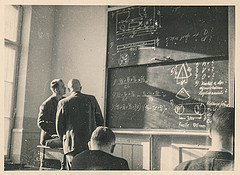### Current coursesIn Spring 2022 I am teaching Math 210b. For a description of this class as well as others, please visit the following link.

### Past courses at Sacramento State

• Spring 2022: Math 210b Section 1.
• Fall 2021: Math 210a Section 1, Math 34 Sections 1 and 2.
• Spring 2021: Math 296c Section 1, Math 45 Sections 11 and 12.
• Fall 2020: Math 110b Section 1, Math 34 Sections 1 and 2.
• Spring 2020: Math 110a Section 3, Math 100 Section 8.
• Fall 2019: Math 117 Section 2, Math 100 Sections 2 and 5.
• Spring 2019: Math 134 Section 1, Math 31 Section 7, Math 45 Sections 2 and 9.
• Fall 2018: Math 110b Section 1, Math 30 Sections 1 and 14.
• Spring 2018: Math 110a Section 3, Math 32 Sections 4 and 6.
• Fall 2017: Math 110b Section 1, Math 45 Section 1.
• Spring 2017: Math 110a Section 3, Math 32 Sections 3 and 4.
• Fall 2016: Math 31 Sections 1 and 3.

Click here for a list of selected talks I have given.